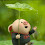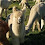### Lab Report on Determination of the Ka of CH3COOH using conductance

The lab report below was submitted as part of the coursework for CM2132 Physical Chemistry. Please do not plagiarise from it as plagiarism might land you into trouble with your university. Do note that my report is well-circulated online and many of my juniors have received soft copies of it. Hence, please exercise prudence while referring to it and, if necessary, cite this webpage.

Aim
To determine the dissociation constant, Ka, of a weak acid (acetic acid, CH3COOH) by measuring the electrical conductance of a series of concentrations of the electrolyte solutions (acetic acid and sodium chloride).
Results and calculation
Conductance of Conductance Water = 0.880 S cm-1
 [CH3COOH] /M Recorded Conductance / µS cm-1 0.00156 66.50 0.00312 91.80 0.00625 133.3 0.01250 191.4 0.02500 273.0 0.05000 376.0
Table 1: Conductance Reading of CH3COOH
When the concentration of acetic acid is 0.00156 M,
actual specific conductance =recorded conductance – conductance of water
= 66.5 -0.880
= 65.62 μScm-1

equivalent conductance , Ʌ = specific conductance of the solution / [CH3COOH]
= 65.62 μS cm-1/0.00156 mol dm-3
= 65.62 x 10-6 S cm-1/ 0.00156 x 10-3 mol cm-3
≈ 42.06 S cm2 mol
= 42060 mScm2equiv-1

The specific conductance and equivalent conductance of the remaining concentrations are calculated using the same method shown above and tabulated in table 2.
 [CH3COOH]/ M √[CH3COOH]/ M½ Recorded Conductance / µS cm-1 Specific Conductance / µS cm-1 Equivalent Conductance, / mS cm2equiv-1 0.00156 0.03950 66.50 65.62 42060 0.00312 0.05586 91.80 90.92 29140 0.00625 0.07906 133.3 132.4 21190 0.01250 0.1118 191.4 190.5 15240 0.02500 0.1581 273.0 272.1 10880 0.05000 0.2236 376.0 375.1 7502
Table 2: The specific conductance and equivalent conductance for acetic acid solution.
Graph 1: Graph of equivalent conductance, /mS cm2 equiv-1 against √[CH3COOH]// M1/2
Similarly, the specific and equivalent conductance of NaCl at different concentrations can be found.
 [NaCl]/ M √[NaCl]/M½ Recorded Conductance / µS cm-1 Specific conductance / μS cm-1 Equivalent Conductance, / mS cm2equiv-1 0.00125 0.03536 169.8 168.9 135100 0.00250 0.05000 334.0 333.1 133200 0.00500 0.07071 656.0 655.1 131000 0.01000 0.10000 1264 1263 126300 0.02000 0.14142 2510 2509 125500 0.04000 0.20000 4950 4949 123700 0.06000 0.24495 7100 7099 118300 0.08000 0.28284 9510 9510 118900
Table 3: The specific conductance and equivalent conductance of NaCl
Graph 2: Equivalent Conductance / mS cm2 equiv-1 against √[NaCl]/M½
For dilute solutions,  Λ = Λo – D ,
By plotting Λ against , the equivalent conductance at infinite dilution Λo for strong electrolyte NaCl can be obtained from the graph.
From graph 2, since the y-intercept                   = 135660, Λo NaCl    =135660 mScm2equiv-1
Λ0HR = Λ0HCl0NaR0NaCl
In this experiment, the value of equivalent conductance at infinite dilution, Λo of HCl and Na(CH3COO) are not determined. Hence, the literature values at 250C will be used.
CH3COONa          = 91.00 S cm2 equiv-1
HCl                         = 426.16 S cm2 equiv-1

Using the equation, Λo CH3COOH    = Λo HCl + Λo CH3COO-Na+ - Λo NaCl
= 426.16 + 91.00 – 135.66
= 383.50 S cm2 equiv-1
= 383500 mS cm2 equiv-1
When [CH3COOH] = 0.00156M,
Λ CH3COOH                      = 42060 mScm2equiv-1
Degree of dissociation, α              = Λ /Λo =
= 0.1097

Apparent dissociation constant, K   = α2C / (1 – α) = (0.10962 x 0.00156) / (1 – 0.1096) 2.108 x 10-5 M

Similar calculations were repeated for various CH3COOH concentrations and the results were tabulated in Table 4 below.
 [CH3COOH]/M Equivalent Conductance, / mS cm2equiv-1 Degree of dissociation, α Apparent dissociation constant, K/ 10-5 M 0.00156 42060 0.1097 1.962 0.00312 29140 0.07598 1.908 0.00625 21190 0.05525 1.880 0.01250 15240 0.03974 1.854 0.02500 10880 0.02837 1.781 0.05000 7502 0.01956 1.850
Table 4: apparent dissociation constant of acetic acid at various concentrations
Experimental value of Ka
= Mean value of K at various concentration of CH3COOH
=
1.873 × 10-5 M
Literature value of Ka of CH3COOH = 1.752 x 10-5 M
Percentage discrepancy =  6.91 %
Discussion
Conductance

The movement of ions through its solution to electrodes is known as conductance. When electrodes are placed in a solution, the cations are attracted to the negatively-charged cathode while the anions move towards the positively-charged anode. This conductance may be affected by changes in ionic concentration and differs between compounds.

For a strong electrolyte, there is complete dissociation into mobile ions. At infinite dilution, the distance between neighboring ions is significant; therefore, only the effect of the applied electric field is experienced by individual ions. However, in a concentrated solution, each ion is surrounded by other ions. The ions are close enough to be influenced by both the electric field applied by the electrodes as well as that by their surrounding ions.

In this experiment, the conductance of NaCl and CH3COOH is measured. NaCl, being an ionic salt, dissociates completely in water to produce Na+ and Cl- ions. Due to a high concentration of mobile ions, it has significant conductance. On the other hand, CH3COOH is a weak acid which only partially ionises in water; hence, its conductance is weaker than that of NaCl.

Kohlrausch’s law

For dilute solutions of strong electrolytes, the equivalent conductance is a linear function of the square root of the concentration. This is in accordance to Kohlrausch’s Square Root Law, Ʌ=Ʌ0 - D   where Ʌ is the conductance of the salt, C, its concentration and D, a function of the valence of the salt.

At infinite dilution, the equivalent conductance of strong electrolyte, NaCl approaches a definite value (graph 2). The extrapolation of graph 2 allows the conductance at infinite dilution, Ʌ0, to be calculated.

However, this may not be done for a weak electrolyte. In the case of CH3COOH, the equivalent conductance at infinite dilution cannot be extrapolated to a definite value. From graph 1, it can be observed that the gradient of the curve varying even at very low concentrations. Thus, for the weak CH3COOH acid, the value of Ʌ may be obtained from a knowledge of the values for Ʌ0 for HCl, NaCl and Na+CH3COO-, according to the equation, Λo CH3COOH = Λo HCl + Λo CH3COO-Na+ - Λo NaCl.

After calculating the conductance of the acid, its dissociation constant may be determined. Weak electrolytes such as acetic acid, do not dissociate completely in solution. Instead, there is an equilibrium between ions and associated electrolyte.
CH3COOH + H2O ↔ CH3COO- + H3O+
Equilibrium concentration   c(1-a)                        ca          ca
Ka =  =  =
Hence, according to the above equation, the acid dissociation constant may be determined.
Sources of discrepancy and handling of experiment
 experimental literature Percentage discrepancy Λo NaCl 135.66 S cm2equiv-1 126.45 S cm2 equiv-1 7.28 % Λo CH3COOH 383.50 S cm2 equiv-1 390.71 S cm2 equiv-1 7.21 % Ka of CH3COOH 1.873 × 10-5 M 1.752 x 10-5 M 6.91 %

As seen from the table above, the experimental values of Λo for NaCl and CH3COOH differ very slightly from their respective literature values. Likewise, the discrepancy between experimental and theoretical dissociation constants of acetic acid is not significant.

Reasons for these deviations may be attributed to the different temperatures at which the literature and experimental values are recorded; the literature values are recorded at the temperature of 25oC while the experimental values were taken around 28oC. Variation in temperature affects the position of the equilibrium; this causes Ka to be a temperature-dependent constant. To reduce discrepancy between the observed and experimental values, a water bath at 25oC could be used.
Also, the solutions should be insulated during the preparation and measurement of the conductance. This minimizes the fluctuations in temperature, hence allowing a more accurate Ka value to be determined.

Conductance  water
Ordinary distilled water is not suitable for use in this experiment as it has a high conductance, usually because of dissolved CO2 gas from the air. Likewise, tap water, with dissolved ions, is unsuitable for use in this experiment.

The conductance water is therefore deionised by passing the water through an ion-exchange resin. It should have conductance of less than 5 × 10-6 siemens. If the conductance of the water is significant, it may mask the conductance of the solutions and reduces the accuracy of their measured conductance. Prior to the experiment, the conductance of water was measured to be 0.88 x 10-6 siemens, which is an acceptable reading.

Experimental techniques

To prepare the various concentrations of solutions, successive dilutions were carried out carefully.  This must be done with utmost precision: should the concentration of a preceding solution be wrongly prepared, this will result in a propagation of errors in the concentrations of successive solutions, thereby further leading to inaccurately measured conductance for all these successive solutions.
After the preparation of the solutions, conductivity measurements are carried out with a dip cell. When not in use, the dip cell is placed in a beaker filled with deionized water to ensure it is reasonably stowed.

Prior to each measurement, the electrodes on the dip cell are rinsed a few times with a dropper containing the solution to be tested. This displaces any residual ions that may be on it. For the same reason, the beaker is also rinsed several times with the solution for which conductance is to be measured.
Time is allowed for the solution to equilibrate before its conductance is measured. Also, a magnetic stirrer should be place in the beaker containing the solution to be measured; this ensures even mixing such that a more representative conductance may be recorded.

Conclusion
The equivalent conductance of strong electrolyte sodium chloride at infinite dilution is 136 S cm2 equiv-1 and that of acetic acid is 384 S cm2 equiv-1. The experimentally determined value of dissociation constant, K of acetic acid is 1.87 × 10-5 M.

References

 Appelo and Postma. Specific Conductance: how to calculate, to use and the pitfalls. Geochemistry, groundwater and pollution, 2nd ed.

 Quid United, Protein Crystallography. Kohlrausch’s law. Article retrieved on 20 Feb 2012:

 Anonymous. Acid dissociation constant. Article retrieved on 22 Feb 2012: <

1.you're welcome :)

2.It really helped me...thanks for your hardwork

3.Really needed this, thanks

4.this lab is calculation heavy, plus, i have to cite reference. sight, my life college student ain't that smooth.

1.it's okay, college will be over quickly and you'd (most likely) miss the college days.

5.where did you get the literature values

6.7.Hard working